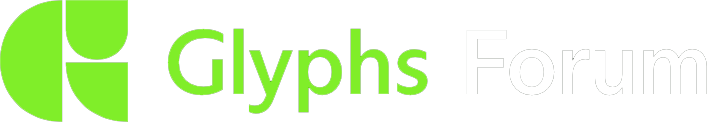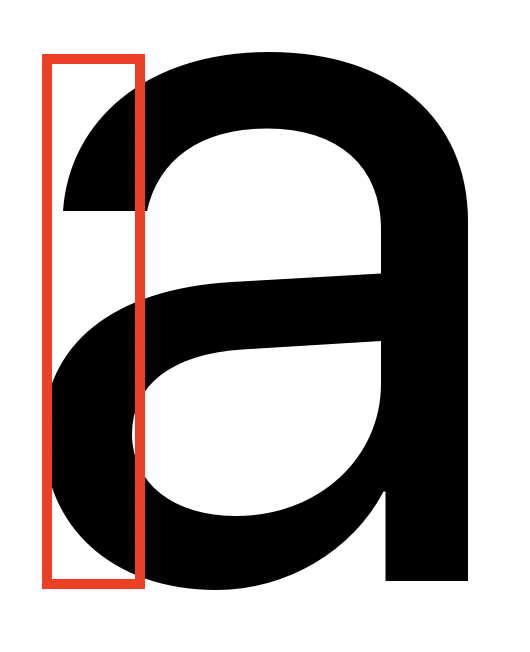# Get area of part of glyph

Hi there,
Is there a way to get the “black” area of a given part of a glyph. Please see attached image exampleYou can rasterize the image and then count the black pixels. Or iterate all coordinates in that rect and test each point:

``````bezierPath = layer.bezierPath
bezierPath.containsPoint_(point)
``````

@GeorgSeifert
Thanks a lot.
I can’t figure out the logic in the coordinates in this way.

I tried completeBezierPath.containsPoint_(point) and that seems to work…

Am I missing something?

completeBezierPath is fine.

I put together a snippet:

``````left = 100
right = 200
top = int(Layer.ascender)
bottom = int(Layer.descender)
steps = 2
path = Layer.bezierPath
white = 0
black = 0
for x in range(left, right, steps):
for y in range(bottom, top, steps):
if path.containsPoint_(NSMakePoint(x, y)):
black += 1
else:
white += 1
print("black", black, "white", white)
``````

If you need to run that for more than one glyph at a time, you might increase the steps otherwise it might take quite long.

Thanks a lot for your help!

What I would do is randomize the x and y of the measure point, calculate a black/white ratio, and keep iterating until the difference to the most recently recorded ratio falls under a threshold. Either you will reach that threshold after you iterated enough times (e.g. 1000 times), or much earlier when the ratio is not changing anymore (e.g. in a completely black area). Do not break off before collecting a minimum amount of measurement points, e.g. 50.

Nice idea. But isn’t there a risk that the randomisation will result in the same coordinates, or is the chance too small? Or do I misunderstand your suggestion?

Same measurement coordinates? Not if you seed first.